Elementary Algebra

# 1.1Introduction to Whole Numbers

Elementary Algebra1.1 Introduction to Whole Numbers

### Learning Objectives

By the end of this section, you will be able to:

• Use place value with whole numbers
• Identify multiples and and apply divisibility tests
• Find prime factorizations and least common multiples

### Be Prepared 1.1

A more thorough introduction to the topics covered in this section can be found in Prealgebra in the chapters Whole Numbers and The Language of Algebra.

As we begin our study of elementary algebra, we need to refresh some of our skills and vocabulary. This chapter will focus on whole numbers, integers, fractions, decimals, and real numbers. We will also begin our use of algebraic notation and vocabulary.

### Use Place Value with Whole Numbers

The most basic numbers used in algebra are the numbers we use to count objects in our world: 1, 2, 3, 4, and so on. These are called the counting numbers. Counting numbers are also called natural numbers. If we add zero to the counting numbers, we get the set of whole numbers.

Counting Numbers: 1, 2, 3, …

Whole Numbers: 0, 1, 2, 3, …

The notation “…” is called ellipsis and means “and so on,” or that the pattern continues endlessly.

We can visualize counting numbers and whole numbers on a number line (see Figure 1.2).

Figure 1.2 The numbers on the number line get larger as they go from left to right, and smaller as they go from right to left. While this number line shows only the whole numbers 0 through 6, the numbers keep going without end.

### Manipulative Mathematics

Doing the Manipulative Mathematics activity “Number Line-Part 1” will help you develop a better understanding of the counting numbers and the whole numbers.

Our number system is called a place value system, because the value of a digit depends on its position in a number. Figure 1.3 shows the place values. The place values are separated into groups of three, which are called periods. The periods are ones, thousands, millions, billions, trillions, and so on. In a written number, commas separate the periods.

Figure 1.3 The number 5,278,194 is shown in the chart. The digit 5 is in the millions place. The digit 2 is in the hundred-thousands place. The digit 7 is in the ten-thousands place. The digit 8 is in the thousands place. The digit 1 is in the hundreds place. The digit 9 is in the tens place. The digit 4 is in the ones place.

### Example 1.1

In the number 63,407,218, find the place value of each digit:

1. 7
2. 0
3. 1
4. 6
5. 3

### Try It 1.1

For the number 27,493,615, find the place value of each digit:

2  1  4  7  5

### Try It 1.2

For the number 519,711,641,328, find the place value of each digit:

9  4  2  6  7

When you write a check, you write out the number in words as well as in digits. To write a number in words, write the number in each period, followed by the name of the period, without the s at the end. Start at the left, where the periods have the largest value. The ones period is not named. The commas separate the periods, so wherever there is a comma in the number, put a comma between the words (see Figure 1.4). The number 74,218,369 is written as seventy-four million, two hundred eighteen thousand, three hundred sixty-nine.

Figure 1.4

### How To

#### Name a Whole Number in Words.

1. Step 1. Start at the left and name the number in each period, followed by the period name.
2. Step 2. Put commas in the number to separate the periods.
3. Step 3. Do not name the ones period.

### Example 1.2

Name the number 8,165,432,098,710 using words.

### Try It 1.3

Name the number $9,258,137,904,0619,258,137,904,061$ using words.

### Try It 1.4

Name the number $17,864,325,619,00417,864,325,619,004$ using words.

We are now going to reverse the process by writing the digits from the name of the number. To write the number in digits, we first look for the clue words that indicate the periods. It is helpful to draw three blanks for the needed periods and then fill in the blanks with the numbers, separating the periods with commas.

### How To

#### Write a Whole Number Using Digits.

1. Step 1. Identify the words that indicate periods. (Remember, the ones period is never named.)
2. Step 2. Draw three blanks to indicate the number of places needed in each period. Separate the periods by commas.
3. Step 3. Name the number in each period and place the digits in the correct place value position.

### Example 1.3

Write nine billion, two hundred forty-six million, seventy-three thousand, one hundred eighty-nine as a whole number using digits.

### Try It 1.5

Write the number two billion, four hundred sixty-six million, seven hundred fourteen thousand, fifty-one as a whole number using digits.

### Try It 1.6

Write the number eleven billion, nine hundred twenty-one million, eight hundred thirty thousand, one hundred six as a whole number using digits.

In 2013, the U.S. Census Bureau estimated the population of the state of New York as 19,651,127. We could say the population of New York was approximately 20 million. In many cases, you don’t need the exact value; an approximate number is good enough.

The process of approximating a number is called rounding. Numbers are rounded to a specific place value, depending on how much accuracy is needed. Saying that the population of New York is approximately 20 million means that we rounded to the millions place.

### Example 1.4

#### How to Round Whole Numbers

Round 23,658 to the nearest hundred.

### Try It 1.7

Round to the nearest hundred: $17,852.17,852.$

### Try It 1.8

Round to the nearest hundred: $468,751.468,751.$

### How To

#### Round Whole Numbers.

1. Step 1. Locate the given place value and mark it with an arrow. All digits to the left of the arrow do not change.
2. Step 2. Underline the digit to the right of the given place value.
3. Step 3.
Is this digit greater than or equal to 5?
• Yes–add $11$ to the digit in the given place value.
• No–do not change the digit in the given place value.
4. Step 4. Replace all digits to the right of the given place value with zeros.

### Example 1.5

Round $103,978103,978$ to the nearest:

1. hundred
2. thousand
3. ten thousand

### Try It 1.9

Round 206,981 to the nearest: hundred thousand ten thousand.

### Try It 1.10

Round 784,951 to the nearest: hundred thousand ten thousand.

### Identify Multiples and Apply Divisibility Tests

The numbers 2, 4, 6, 8, 10, and 12 are called multiples of 2. A multiple of 2 can be written as the product of a counting number and 2.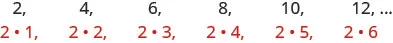Similarly, a multiple of 3 would be the product of a counting number and 3.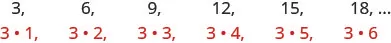We could find the multiples of any number by continuing this process.

### Manipulative Mathematics

Doing the Manipulative Mathematics activity “Multiples” will help you develop a better understanding of multiples.

Table 1.1 shows the multiples of 2 through 9 for the first 12 counting numbers.

Counting Number 1 2 3 4 5 6 7 8 9 10 11 12
Multiples of 2 2 4 6 8 10 12 14 16 18 20 22 24
Multiples of 3 3 6 9 12 15 18 21 24 27 30 33 36
Multiples of 4 4 8 12 16 20 24 28 32 36 40 44 48
Multiples of 5 5 10 15 20 25 30 35 40 45 50 55 60
Multiples of 6 6 12 18 24 30 36 42 48 54 60 66 72
Multiples of 7 7 14 21 28 35 42 49 56 63 70 77 84
Multiples of 8 8 16 24 32 40 48 56 64 72 80 88 96
Multiples of 9 9 18 27 36 45 54 63 72 81 90 99 108
Multiples of 10 10 20 30 40 50 60 70 80 90 100 110 120
Table 1.1

### Multiple of a Number

A number is a multiple of n if it is the product of a counting number and n.

Another way to say that 15 is a multiple of 3 is to say that 15 is divisible by 3. That means that when we divide 3 into 15, we get a counting number. In fact, $15÷315÷3$ is 5, so 15 is $5·3.5·3.$

### Divisible by a Number

If a number m is a multiple of n, then m is divisible by n.

Look at the multiples of 5 in Table 1.1. They all end in 5 or 0. Numbers with last digit of 5 or 0 are divisible by 5. Looking for other patterns in Table 1.1 that shows multiples of the numbers 2 through 9, we can discover the following divisibility tests:

### Divisibility Tests

A number is divisible by:

• 2 if the last digit is 0, 2, 4, 6, or 8.
• 3 if the sum of the digits is divisible by 3.
• 5 if the last digit is 5 or 0.
• 6 if it is divisible by both 2 and 3.
• 10 if it ends with 0.

### Example 1.6

Is 5,625 divisible by 2? By 3? By 5? By 6? By 10?

### Try It 1.11

Determine whether 4,962 is divisible by 2, by 3, by 5, by 6, and by 10.

### Try It 1.12

Determine whether 3,765 is divisible by 2, by 3, by 5, by 6, and by 10.

### Find Prime Factorizations and Least Common Multiples

In mathematics, there are often several ways to talk about the same ideas. So far, we’ve seen that if m is a multiple of n, we can say that m is divisible by n. For example, since 72 is a multiple of 8, we say 72 is divisible by 8. Since 72 is a multiple of 9, we say 72 is divisible by 9. We can express this still another way.

Since $8·9=72,8·9=72,$ we say that 8 and 9 are factors of 72. When we write $72=8·9,72=8·9,$ we say we have factored 72.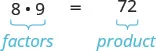Other ways to factor 72 are $1·72,2·36,3·24,4·18,and6·12.1·72,2·36,3·24,4·18,and6·12.$ Seventy-two has many factors: 1, 2, 3, 4, 6, 8, 9, 12, 18, 36, and 72.

### Factors

If $a·b=m,a·b=m,$ then a and b are factors of m.

Some numbers, like 72, have many factors. Other numbers have only two factors.

### Manipulative Mathematics

Doing the Manipulative Mathematics activity “Model Multiplication and Factoring” will help you develop a better understanding of multiplication and factoring.

### Prime Number and Composite Number

A prime number is a counting number greater than 1, whose only factors are 1 and itself.

A composite number is a counting number that is not prime. A composite number has factors other than 1 and itself.

### Manipulative Mathematics

Doing the Manipulative Mathematics activity “Prime Numbers” will help you develop a better understanding of prime numbers.

The counting numbers from 2 to 19 are listed in Figure 1.5, with their factors. Make sure to agree with the “prime” or “composite” label for each!

Figure 1.5

The prime numbers less than 20 are 2, 3, 5, 7, 11, 13, 17, and 19. Notice that the only even prime number is 2.

A composite number can be written as a unique product of primes. This is called the prime factorization of the number. Finding the prime factorization of a composite number will be useful later in this course.

### Prime Factorization

The prime factorization of a number is the product of prime numbers that equals the number.

To find the prime factorization of a composite number, find any two factors of the number and use them to create two branches. If a factor is prime, that branch is complete. Circle that prime!

If the factor is not prime, find two factors of the number and continue the process. Once all the branches have circled primes at the end, the factorization is complete. The composite number can now be written as a product of prime numbers.

Factor 48.

### Try It 1.13

Find the prime factorization of 80.

### Try It 1.14

Find the prime factorization of 60.

### How To

#### Find the Prime Factorization of a Composite Number.

1. Step 1. Find two factors whose product is the given number, and use these numbers to create two branches.
2. Step 2. If a factor is prime, that branch is complete. Circle the prime, like a bud on the tree.
3. Step 3. If a factor is not prime, write it as the product of two factors and continue the process.
4. Step 4. Write the composite number as the product of all the circled primes.

### Example 1.8

Find the prime factorization of 252.

### Try It 1.15

Find the prime factorization of 126.

### Try It 1.16

Find the prime factorization of 294.

One of the reasons we look at multiples and primes is to use these techniques to find the least common multiple of two numbers. This will be useful when we add and subtract fractions with different denominators. Two methods are used most often to find the least common multiple and we will look at both of them.

The first method is the Listing Multiples Method. To find the least common multiple of 12 and 18, we list the first few multiples of 12 and 18: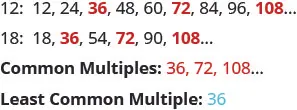Notice that some numbers appear in both lists. They are the common multiples of 12 and 18.

We see that the first few common multiples of 12 and 18 are 36, 72, and 108. Since 36 is the smallest of the common multiples, we call it the least common multiple. We often use the abbreviation LCM.

### Least Common Multiple

The least common multiple (LCM) of two numbers is the smallest number that is a multiple of both numbers.

The procedure box lists the steps to take to find the LCM using the prime factors method we used above for 12 and 18.

### How To

#### Find the Least Common Multiple by Listing Multiples.

1. Step 1. List several multiples of each number.
2. Step 2. Look for the smallest number that appears on both lists.
3. Step 3. This number is the LCM.

### Example 1.9

Find the least common multiple of 15 and 20 by listing multiples.

### Try It 1.17

Find the least common multiple by listing multiples: 9 and 12.

### Try It 1.18

Find the least common multiple by listing multiples: 18 and 24.

Our second method to find the least common multiple of two numbers is to use The Prime Factors Method. Let’s find the LCM of 12 and 18 again, this time using their prime factors.

### Example 1.10

#### How to Find the Least Common Multiple Using the Prime Factors Method

Find the Least Common Multiple (LCM) of 12 and 18 using the prime factors method.

Notice that the prime factors of 12 $(2·2·3)(2·2·3)$ and the prime factors of 18 $(2·3·3)(2·3·3)$ are included in the LCM $(2·2·3·3).(2·2·3·3).$ So 36 is the least common multiple of 12 and 18.

By matching up the common primes, each common prime factor is used only once. This way you are sure that 36 is the least common multiple.

### Try It 1.19

Find the LCM using the prime factors method: 9 and 12.

### Try It 1.20

Find the LCM using the prime factors method: 18 and 24.

### How To

#### Find the Least Common Multiple Using the Prime Factors Method.

1. Step 1. Write each number as a product of primes.
2. Step 2. List the primes of each number. Match primes vertically when possible.
3. Step 3. Bring down the columns.
4. Step 4. Multiply the factors.

### Example 1.11

Find the Least Common Multiple (LCM) of 24 and 36 using the prime factors method.

### Try It 1.21

Find the LCM using the prime factors method: 21 and 28.

### Try It 1.22

Find the LCM using the prime factors method: 24 and 32.

### Section 1.1 Exercises

#### Practice Makes Perfect

Use Place Value with Whole Numbers

In the following exercises, find the place value of each digit in the given numbers.

1.

51,493
1
4
9
5
3

2.

87,210
2
8
0
7
1

3.

164,285
5
6
1
8
2

4.

395,076
5
3
7
0
9

5.

93,285,170
9
8
7
5
3

6.

36,084,215
8
6
5
4
3

7.

7,284,915,860,132
7
4
5
3
0

8.

2,850,361,159,433
9
8
6
4
2

In the following exercises, name each number using words.

9.

1,078

10.

5,902

11.

364,510

12.

146,023

13.

5,846,103

14.

1,458,398

15.

37,889,005

16.

62,008,465

In the following exercises, write each number as a whole number using digits.

17.

four hundred twelve

18.

two hundred fifty-three

19.

thirty-five thousand, nine hundred seventy-five

20.

sixty-one thousand, four hundred fifteen

21.

eleven million, forty-four thousand, one hundred sixty-seven

22.

eighteen million, one hundred two thousand, seven hundred eighty-three

23.

three billion, two hundred twenty-six million, five hundred twelve thousand, seventeen

24.

eleven billion, four hundred seventy-one million, thirty-six thousand, one hundred six

In the following, round to the indicated place value.

25.

Round to the nearest ten.

386 2,931

26.

Round to the nearest ten.

792 5,647

27.

Round to the nearest hundred.

13,748 391,794

28.

Round to the nearest hundred.

28,166 481,628

29.

Round to the nearest ten.

1,492 1,497

30.

Round to the nearest ten.

2,791 2,795

31.

Round to the nearest hundred.

63,994 63,940

32.

Round to the nearest hundred.

49,584 49,548

In the following exercises, round each number to the nearest hundred, thousand, ten thousand.

33.

392,546

34.

619,348

35.

2,586,991

36.

4,287,965

Identify Multiples and Factors

In the following exercises, use the divisibility tests to determine whether each number is divisible by 2, 3, 5, 6, and 10.

37.

84

38.

9,696

39.

75

40.

78

41.

900

42.

800

43.

986

44.

942

45.

350

46.

550

47.

22,335

48.

39,075

Find Prime Factorizations and Least Common Multiples

In the following exercises, find the prime factorization.

49.

86

50.

78

51.

132

52.

455

53.

693

54.

400

55.

432

56.

627

57.

2,160

58.

2,520

In the following exercises, find the least common multiple of the each pair of numbers using the multiples method.

59.

8, 12

60.

4, 3

61.

12, 16

62.

30, 40

63.

20, 30

64.

44, 55

In the following exercises, find the least common multiple of each pair of numbers using the prime factors method.

65.

8, 12

66.

12, 16

67.

28, 40

68.

84, 90

69.

55, 88

70.

60, 72

#### Everyday Math

71.

Writing a Check Jorge bought a car for $24,493. He paid for the car with a check. Write the purchase price in words. 72. Writing a Check Marissa’s kitchen remodeling cost$18,549. She wrote a check to the contractor. Write the amount paid in words.

73.

Buying a Car Jorge bought a car for $24,493. Round the price to the nearest ten hundred thousand; and ten-thousand. 74. Remodeling a Kitchen Marissa’s kitchen remodeling cost$18,549, Round the cost to the nearest ten hundred thousand and ten-thousand.

75.

Population The population of China was 1,339,724,852 on November 1, 2010. Round the population to the nearest billion hundred-million; and million.

76.

Astronomy The average distance between Earth and the sun is 149,597,888 kilometers. Round the distance to the nearest hundred-million ten-million; and million.

77.

Grocery Shopping Hot dogs are sold in packages of 10, but hot dog buns come in packs of eight. What is the smallest number that makes the hot dogs and buns come out even?

78.

Grocery Shopping Paper plates are sold in packages of 12 and party cups come in packs of eight. What is the smallest number that makes the plates and cups come out even?

#### Writing Exercises

79.

Give an everyday example where it helps to round numbers.

80.

If a number is divisible by 2 and by 3 why is it also divisible by 6?

81.

What is the difference between prime numbers and composite numbers?

82.

Explain in your own words how to find the prime factorization of a composite number, using any method you prefer.

#### Self Check

After completing the exercises, use this checklist to evaluate your mastery of the objectives of this section.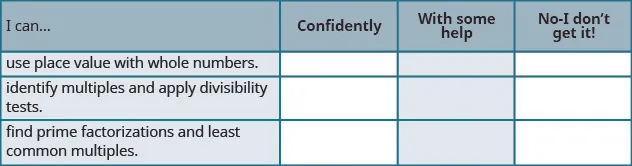If most of your checks were:

…confidently. Congratulations! You have achieved the objectives in this section. Reflect on the study skills you used so that you can continue to use them. What did you do to become confident of your ability to do these things? Be specific.

…with some help. This must be addressed quickly because topics you do not master become potholes in your road to success. In math, every topic builds upon previous work. It is important to make sure you have a strong foundation before you move on. Who can you ask for help? Your fellow classmates and instructor are good resources. Is there a place on campus where math tutors are available? Can your study skills be improved?

…no—I don’t get it! This is a warning sign and you must not ignore it. You should get help right away or you will quickly be overwhelmed. See your instructor as soon as you can to discuss your situation. Together you can come up with a plan to get you the help you need.

Order a print copy

As an Amazon Associate we earn from qualifying purchases.